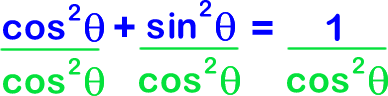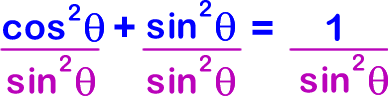Most people easily remember this first identity... But, often times, you'll need to remember the other two when you get to Calculus.

Here's an easy way to derive the next two, starting from the first one:

2) Divide everywhere bySimplifying gives us the second Pythagorean Identity...3) Divide everywhere bySimplifying gives us the third and final Pythagorean Identity...* I always remember that the "co" guys go together! (cotangent and cosecant)